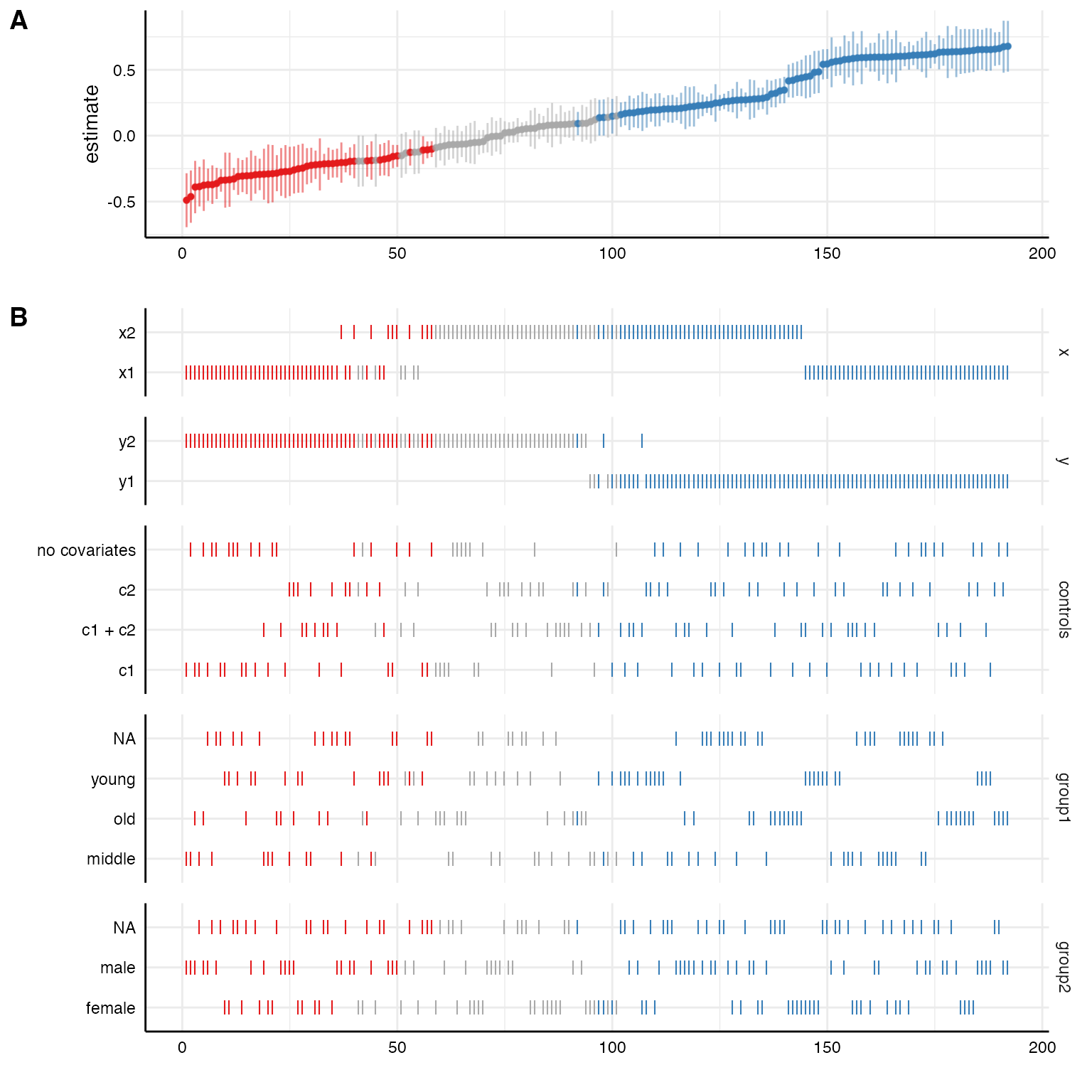This vignette exemplifies different ways to plot the specification curve. For most cases, the function plot_specs() takes care of the overall process. However, more specific customization is possible if we use the more specific functions plot_curve() and plot_choices. Furthermore, we can extend the overall plot with the additional function plot_samplesizes(). All of these functions produce objects of the class ggplot that can be customized further using the grammar of graphics provided by the package ggplot2.

## 1. Run the specification curve analysis

In order to have some data to work with, we run the minimal example included in the package.

library(specr)
library(dplyr)
library(ggplot2)
library(cowplot)

# run spec analysis
results <- run_specs(example_data,
y = c("y1", "y2"),
x = c("x1", "x2"),
model = "lm",
controls = c("c1", "c2"),
subset = list(group1 = unique(example_data$group1), group2 = unique(example_data$group2)))

Let’s quickly get some ideas about the specification curve by using summarise_specs()

summarise_specs(results)
## # A tibble: 1 x 7
##   median   mad   min   max   q25   q75   obs
##    <dbl> <dbl> <dbl> <dbl> <dbl> <dbl> <dbl>
## 1   3.59  4.56 -2.05  9.58  1.03  7.63   123
summarise_specs(results, x)
## # A tibble: 2 x 8
##   x     median   mad    min   max   q25   q75   obs
##   <chr>  <dbl> <dbl>  <dbl> <dbl> <dbl> <dbl> <dbl>
## 1 x1      3.59  4.42 -2.05   9.28 0.510  6.51   123
## 2 x2      4.40  4.84 -0.258  9.58 1.31   7.79   123

We see that it makes quite a difference whether x1 or x2 is used as independent variable.

## 2. A simple way to visualize the results

The simplest way to visualize most of the information contained in the results data frame is by using the plot_specs() function.

plot_specs(results)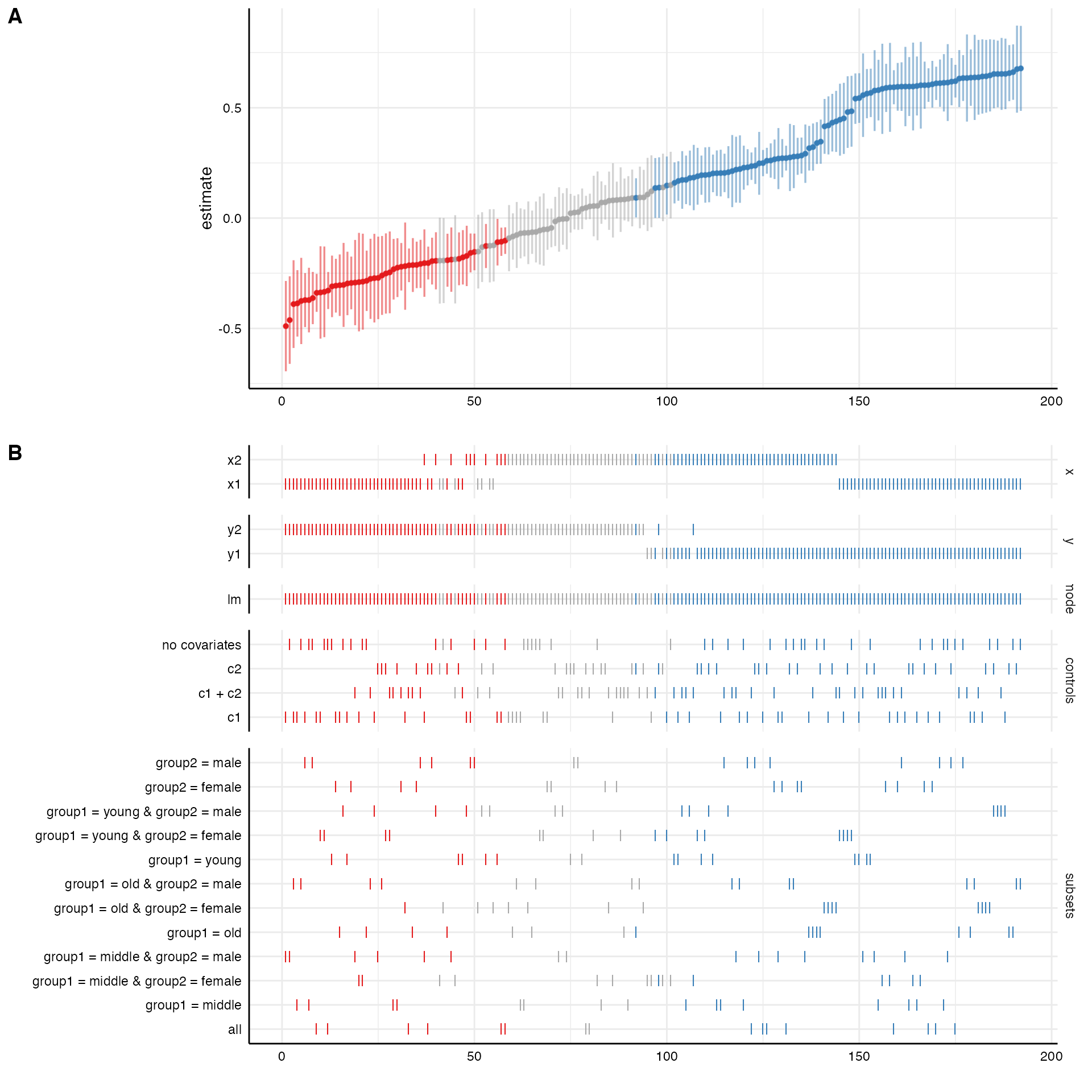We can further customize that function, e.g., by removing unnecessary information (in this case we only specified one model, this analytical choice is hence useless) or by reordering/transforming the analytical choices (and thereby visualize specific contrasts).

plot_specs(results,
choices = c("x", "y", "controls", "subsets"),  # "model is not plotted
rel_heights = c(1, 2))                         # changing relative heights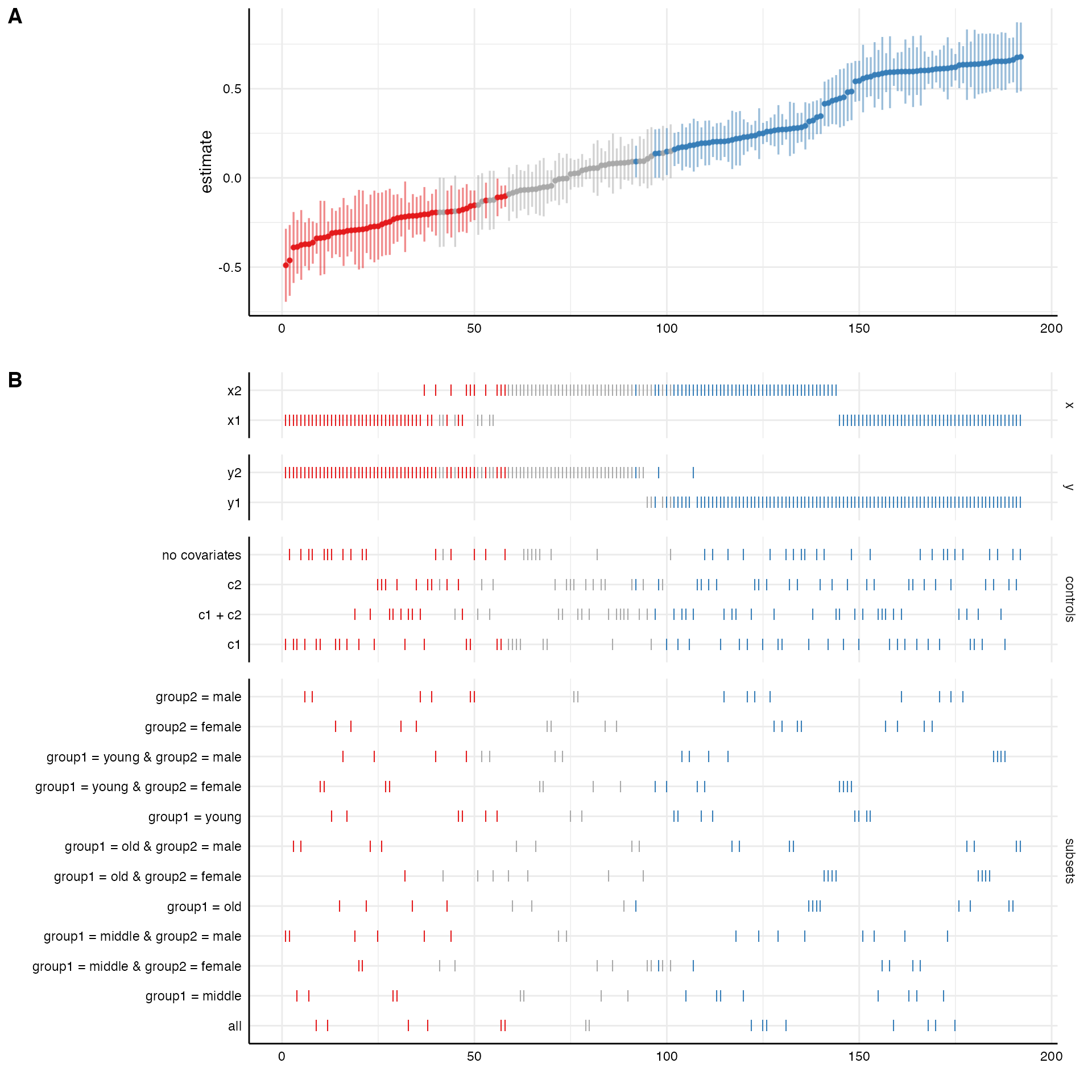# Investigating specific contrasts
results %>%
mutate(group1 = ifelse(grepl("group1 = 0", subsets), "0", "1"),
group2 = ifelse(grepl("group2 = A", subsets), "A", "B & C")) %>%
plot_specs(choices = c("x", "y", "controls", "group1", "group2"))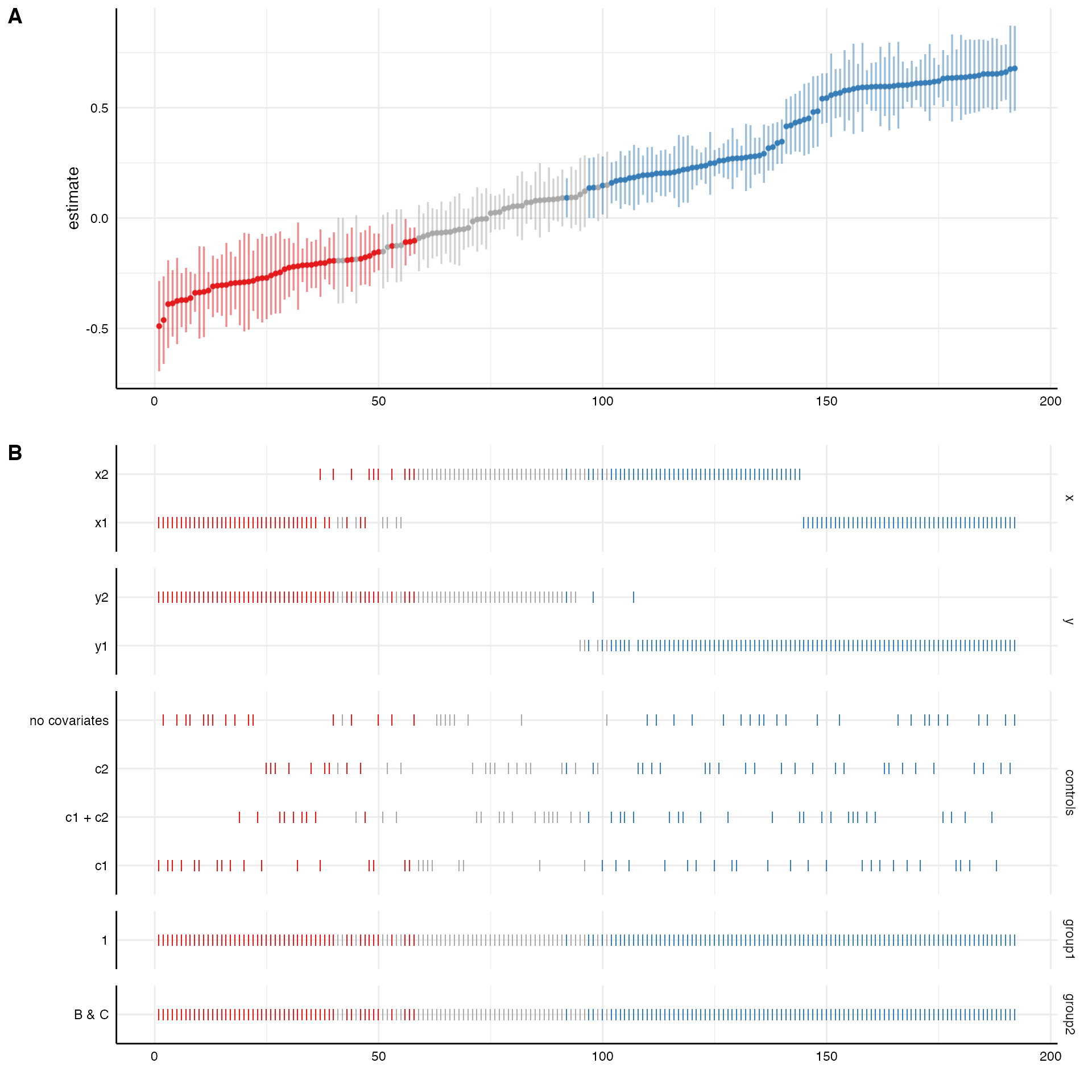## 3. An alternative way to visualize the results

### 3.1. Plot curve and choices seperately

The overall function plot_specs() is not very flexible. Alternatively, we can plot the specification curve and the choice panel individually and bind them together afterwards. This is useful as it allows us to customize and change both individual plots.

# Plot specification curve
p1 <- plot_curve(results,
ci = FALSE,
ribbon = TRUE) +
geom_hline(yintercept = 0,
linetype = "dashed",
color = "black") +
ylim(-8, 14) +
labs(x = "", y = "unstandarized regression coefficient") +
theme_half_open()

# Plot choices
p2 <- plot_choices(results,
choices = c("x", "y", "controls", "subsets")) +
labs(x = "specifications (ranked)") +
theme_half_open() +
theme(strip.text.x = element_blank())

# Combine plots
plot_specs(plot_a = p1,
plot_b = p2,
labels = c("", ""),      # remove plot labels
rel_height = c(2, 2.5))  # adjust relative heights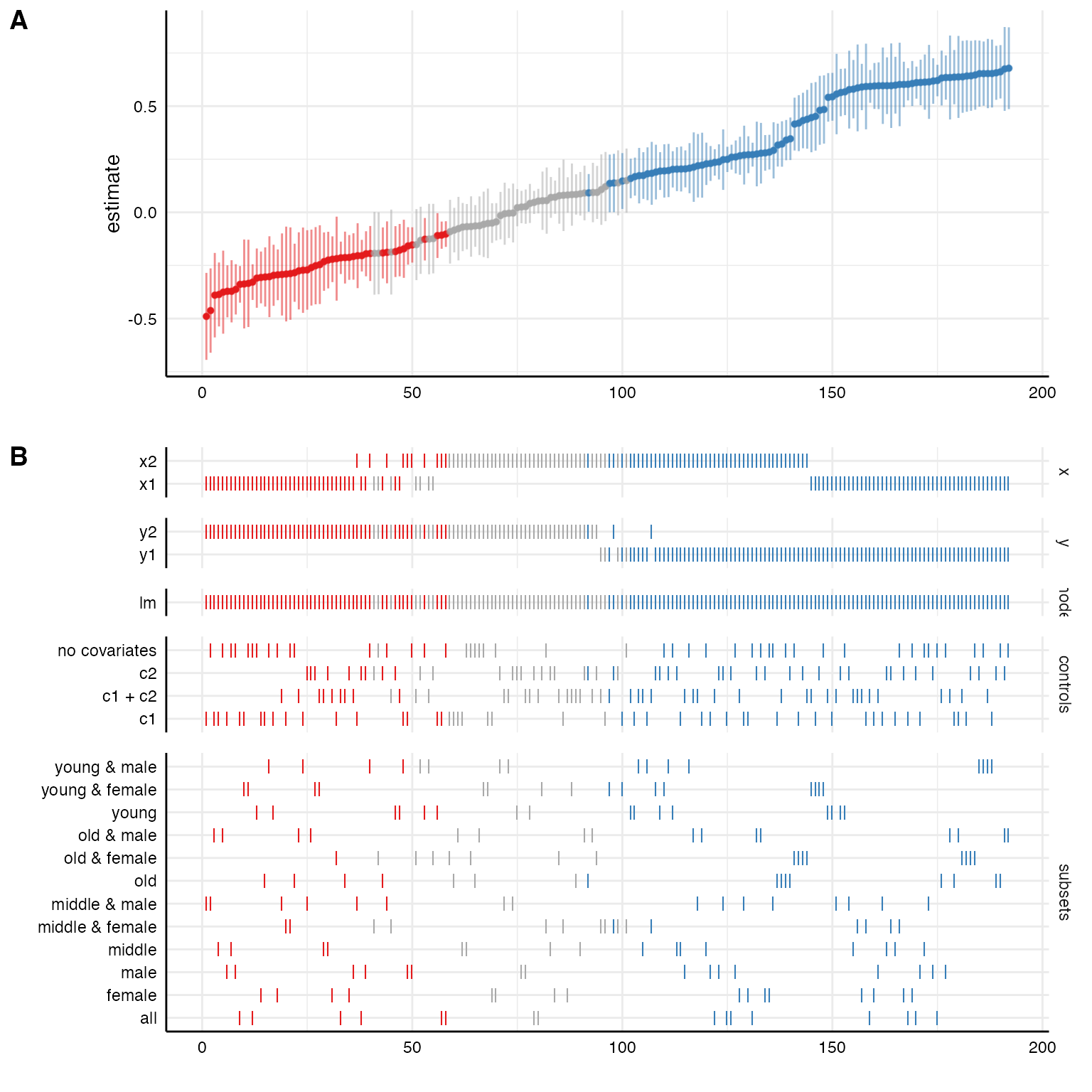### 3.2. Include sample size histogram

By default, we do not know how many participants were included in each specification. If you remove missing values listwise, this may not be a big problem as all models are based on the same subsample. If you have missing values in your dataset and you did not impute them or delete them listwise, we should investigate how many participants were included in each specification. The function plot_samplesizes() provides an additional panel that can then be added to the overall plot using cowplot::plot_grid()

p3 <- plot_samplesizes(results) +
theme_half_open()

# Combine via cowplot
plot_grid(p1, p2, p3,
ncol = 1,
align = "v",
rel_heights = c(1.5, 2, 0.8),
axis = "rbl")